• stl常用函数lower_bound,upper_bound和equal_range函数初识注意事项具体使用说明equal_range函数使用注意事项高级用法 lower_bound,upper_bound和equal_range函数初识 lower_bound.(k) 返回一个迭代器，...


stl常用函数
lower_bound,upper_bound和equal_range函数初识注意事项具体使用说明equal_range函数使用注意事项高级用法

lower_bound,upper_bound和equal_range函数初识
lower_bound.(k)返回一个迭代器，指向第一个值不小于k的元素upper_bound(k)返回一个迭代器，指向第一个值大于k的元素equal_range(k)返回一个迭代器pair,表示值等于k的元素的范围。若k不存在，pair两个成员均等于end()–尾迭代器
上面三个函数多用于容器中使用，但是对于普通的数组也是可以使用的，下面会讲到.如果所查找值在容器中，lower_bound返回的迭代器将指向第一个具有给定值的元素，而upper_bound返回的迭代器指向最后一个匹配给定值的元素之后的位置。如果元素不在容器中，则lower_bound和upper_bound会返回相等的迭代器----指向一个不影响排序的值插入位置因此，用相同的值调用lower_bound和upper_bound会得到一个迭代器的范围,表示具有该关键字的元素范围。当然，这两个操作返回的迭代器可能是容器的尾后迭代器。如果我们查找的元素具有容器中最大值，则此关键字的upper_bound返回尾后迭代器。如果关键字不存在，且大于容器中任何关键字，则lower_bound返回的也是尾后迭代器.

注意事项
lower_bound返回的迭代器可能指向一个具有给定值的元素，但也可能不指向。如果关键字不在容器中，则lower_bound会返回关键字的第一个安全插入点—不影响容器中元素顺序的插入位置如果lower_bound和upper_bound返回相同的迭代器，则给定的关键字不在容器中.

具体使用说明
1.与map容器结合使用，打印map容器中作者英文名字对应为Tom的所有出版书籍.
void test()
{
//注意map容器不能插入重复关键字
map<string, string> author = {
{"Jerry","Jerry's travel"},
{"Boboy","Star Star Star"},
{"Tom","I Love Mouse"},
};
for (auto beg = author.lower_bound("Tom"), end = author.upper_bound("Tom"); beg != end; beg++)
cout << beg->second << endl;
}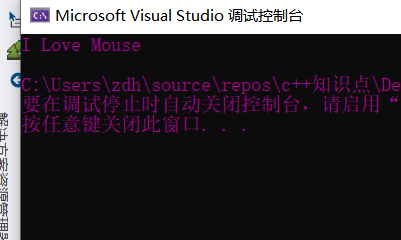2.与vector结合使用，打印vector数组中大于等于5的元素范围
	//这里数组v最好是有序的
vector<int> v = { 1,2,3,4,5,6,7,8 };
for (auto beg = lower_bound(v.begin(), v.end(),5); beg != v.end(); beg++)
cout << *beg <<" " <<ends;
cout << endl;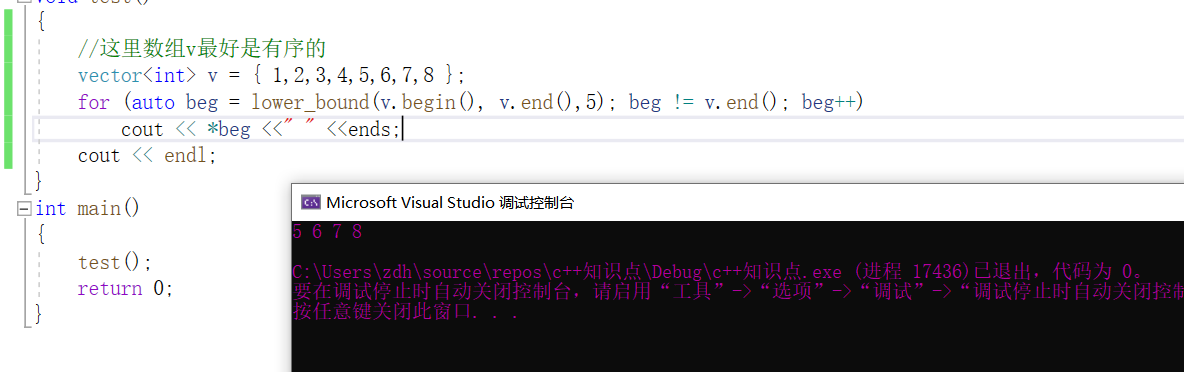注意:数组最好是有序的，不然打印范围是错误的
3,在vector数组中查找存在的元素2
注意:数组必须是有序的，不然查找结果会出错
	//这里数组v最好是有序的
vector<int> v = { 1,2,3,4,5,6,7,8};
auto beg = lower_bound(v.begin(), v.end(), 5), end = upper_bound(v.begin(), v.end(), 5);
cout << *beg <<" " <<ends;
cout << endl<<*end << endl;
cout << endl;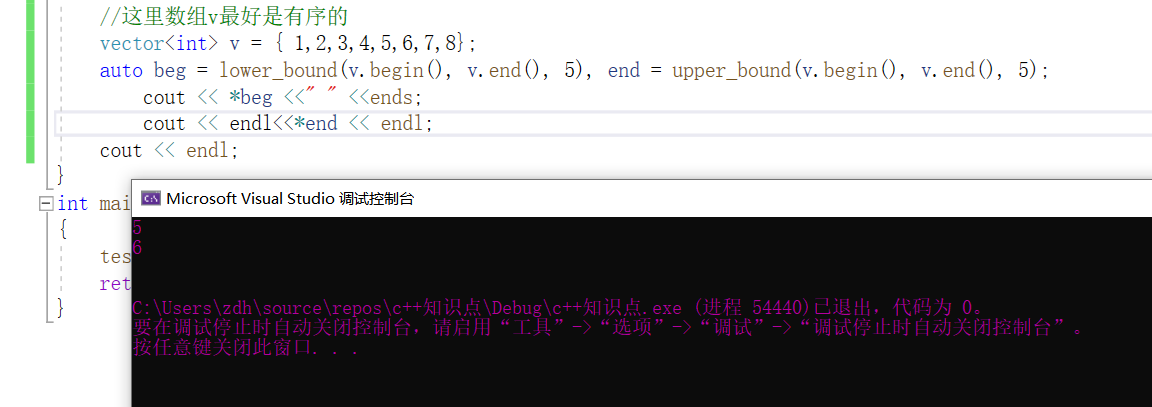注意观察这里beg和end查找到的对应值区别，beg从前往后找到第一个大于等于对应值的元素，而end从后往前查找第一个大于对应值的元素。
4,在vector数组中查找不存在的元素
1.如果查找的不存在元素是4
	//这里数组v最好是有序的
vector<int> v = { 1,2,3,5,6,7,8};
auto beg = lower_bound(v.begin(), v.end(), 4), end = upper_bound(v.begin(), v.end(), 4);
if (beg == v.end())
cout << "beg此时指向尾迭代器" << endl;
else
cout << *beg << endl;
if (end == v.end())
cout << "end此时指向尾迭代器" << endl;
cout << *end << endl;
cout << endl;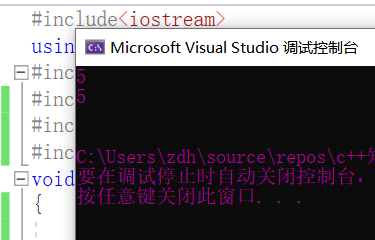2.如果查找的不存在元素是8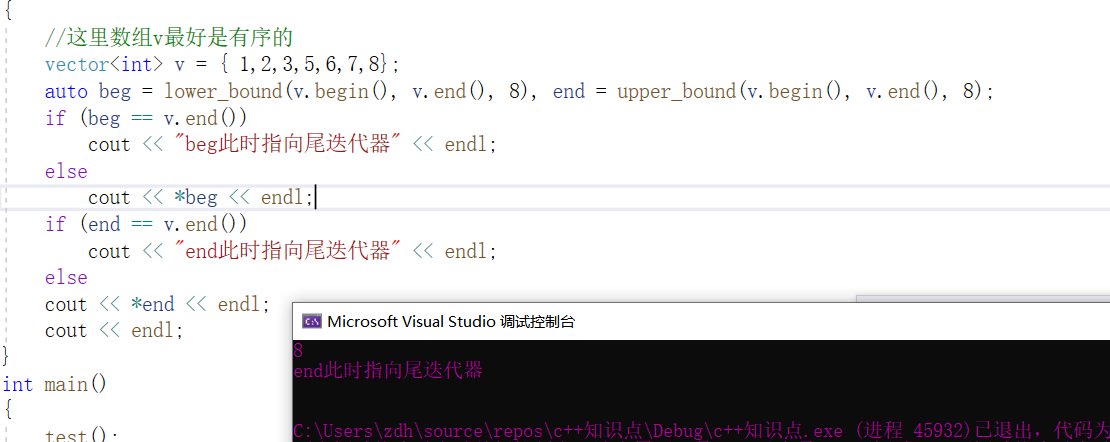3.如果查找的不存在元素是10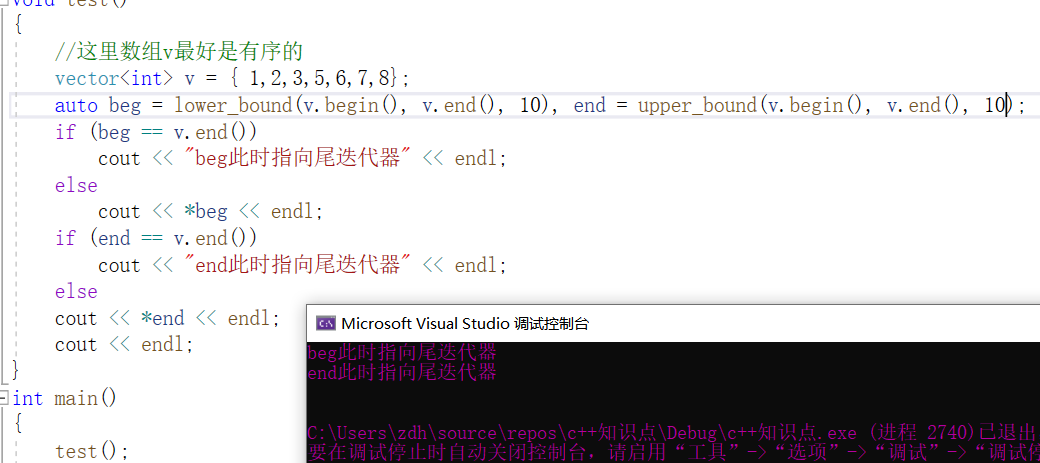4,利用equal_range函数打印map容器中所有关键字为2的元素
void test()
{
multimap<int, int> map = {
{1,0},
{1,520},
{2,4},
{2,3},
{2,6},
{4,5},
{7,8},
{10,22},
};
for (auto beg = map.lower_bound(2), end = map.upper_bound(2); beg!= end; beg++)
cout << beg->second << endl;
}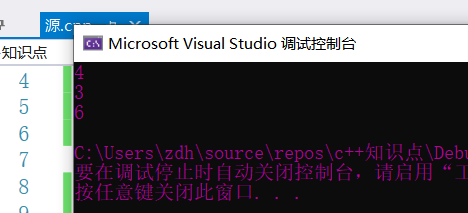equal_range函数使用注意事项
此函数接受一个关键字，返回一个迭代器pair。  若关键字存在，则第一个迭代器指向第一个与关键字匹配的元素，第二个迭代器指向最后一个与关键字匹配的元素之后的位置。  若未找到匹配元素，则两个迭代器都指向关键字可以插入的位置.  其实equal_range返回的pair，此pair的first成员保存的迭代器与lower_bound返回的迭代器是一样的，second保存的迭代器与upper_bound的返回值是一样的。

高级用法
这里先介绍一种高级用法，用lower_bound来简化二分查找算法的写法:
这里顺便也补上lower_bound函数与普通数组结合使用的案例
bool BS(int* arr,int val)
{
auto pos=lower_bound(arr, arr + 8, val);
if (*pos== val)
return true;
return false;
}
int main()
{
int arr = { 1,2,3,4,5,6,7,8 };
cout << "查找指定元素4是否在数组中" << endl;
if (BS(arr, 4))
cout << "查找到指定元素" << endl;
else
cout << "没有此元素" << endl;
return 0;
}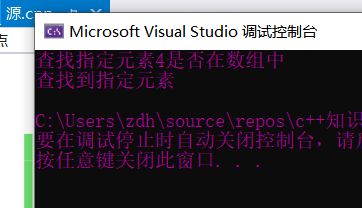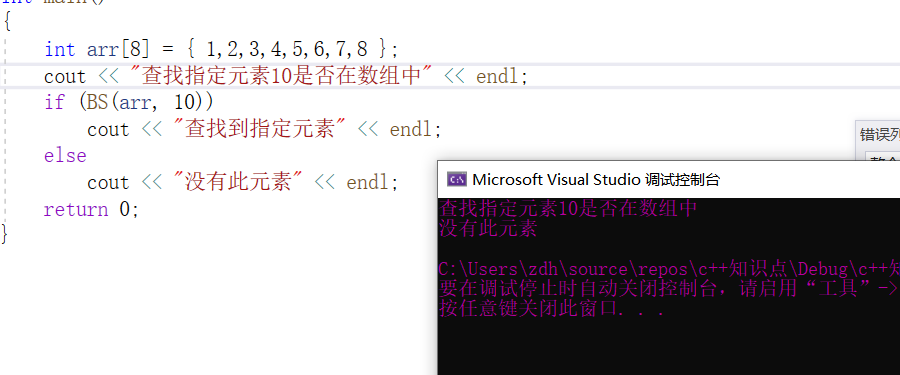展开全文• up = upper_bound(v1.begin(), v1.end(), 3); cout *up ; vector<int> v2 = {0, 1, 5, 3, 2, 1, 4, 7, 6}; vector<int>::iterator lo; lo = lower_bound(v2.begin(), v2.end(), 3); cout *lo ; ...c++ c++ c++
• 头文件：lower_boundupper_bound在头文件algorithm中； 解释：lower_boundupper_bound为二分法查找元素，其时间复杂度为O(log n)。 一、数组中的lower_boundupper_bound 对于一个排序数组 nums{1, 2, 5, 7,...
头文件：lower_bound和upper_bound在头文件algorithm中； 解释：lower_bound和upper_bound为二分法查找元素，其时间复杂度为O(log n)。
一、数组中的lower_bound和upper_bound
对于一个排序数组 nums{1, 2, 5, 7, 9};
（1） int i = lower_bound(nums, nums + n, val) - nums;
函数解释：lower_bound函数返回数组 nums 中大于等于 val 的第一个元素的地址，若 nums 中的元素均小于 val 则返回尾后地址。
#include<iostream>
#include<algorithm>
using namespace std;

int main()
{
const int n = 5;
int nums[n]{ 1,2,5,7,9 };
int i = lower_bound(nums, nums + n, 6) - nums;//大于等于6
cout << i << endl;//i=3
int j = lower_bound(nums, nums + n, 10) - nums;
cout << j << endl;//j=5

system("pause");
return 0;
}

（2） int i = upper_bound(nums, nums + n, val) - nums;
函数解释：upper_bound函数返回数组 nums 中大于 val 的第一个元素的地址，若 nums 中的元素均小于等于 val 则返回尾后地址。
#include<iostream>
#include<algorithm>
using namespace std;

int main()
{
const int n = 5;
int nums[n]{ 1,2,5,7,9 };
int i = upper_bound(nums, nums + n, 6) - nums;//大于6
cout << i << endl;//i=3
int j = upper_bound(nums, nums + n, 9) - nums;
cout << j << endl;//j=5

system("pause");
return 0;
}

二、STL中的lower_bound和upper_bound
用法和数组中的用法基本一样，不同之处在于写法和返回值，STL返回值为迭代器，写法如下：
（1）vector
#include<iostream>
#include<algorithm>
#include<vector>
using namespace std;

int main()
{
vector<int> vec{ 1,2,5,7,9 };//有序数组
vector<int>::iterator it1 = lower_bound(vec.begin(), vec.end(), 9);
bool flag1 = it1 == vec.end() - 1;
cout << flag1 << endl;//it1指向最后一个元素的迭代器
vector<int>::iterator it2 = upper_bound(vec.begin(), vec.end(), 9);
bool flag2 = it2 == vec.end();
cout << flag2 << endl;//it2为尾后迭代器

system("pause");
return 0;
}

（2）set
#include<iostream>
#include<algorithm>
#include<set>
using namespace std;

int main()
{
set<int> s{ 1,2,1,9,7 };//原本就有序
set<int>::iterator it1 = s.lower_bound(2);
cout << *it1 << endl;//*it1=2
set<int>::iterator it2 = s.upper_bound(2);
cout << *it2 << endl;//*it2=7

system("pause");
return 0;
}

（3）map
#include<iostream>
#include<algorithm>
#include<map>
using namespace std;

int main()
{
map<int, int> m{ {1,2},{2,2},{1,2},{9,2},{7,2} };//有序
map<int, int>::iterator it1 = m.lower_bound(2);
cout << it1->first << endl;//it1->first=2
map<int, int>::iterator it2 = m.upper_bound(2);
cout << it2->first << endl;//it2->first=7

system("pause");
return 0;
}

展开全文c++ stl 二分法
• lower_boundupper_bound是C++ STL中提供的函数。操作对象可以是vector、set以及map。 upper_bound(i)：返回map中第一个大于key的迭代器指针 lower_bound(i)：返回map中第一个大于或等于key的迭代器指针 vector ...
lower_bound和upper_bound是C++ STL中提供的函数。操作对象可以是vector、set以及map。
upper_bound(i)：返回map中第一个大于key的迭代器指针
lower_bound(i)：返回map中第一个大于或等于key的迭代器指针
vector
#include<iostream>
#include<algorithm>
#include<vector>
using namespace std;

int main()
{
int a[]={1,2,4,4,2,1,1,2};

vector<int>v(a,a+8);
sort(v.begin(),v.end());

vector<int>::iterator it1,it2;

it1=lower_bound(v.begin(),v.end(),2);
it2=upper_bound(v.begin(),v.end(),2);

//int x=it1-v.begin();cout<<"x="<<x<<endl;
//int y=it2-v.begin();cout<<"y="<<x<<endl;

cout<<"Pos_lower->"<<(it1-v.begin())<<endl;
cout<<"Pos_upper->"<<(it2-v.begin())<<endl;

return 0;
}

set
#include<iostream>
#include<set>
using namespace std;

int main()
{
set<int>s;
set<int>::iterator it1,it2,it;

for(int i=1;i<10;++i) s.insert(i);

it1=s.lower_bound(3);
it2=s.upper_bound(6);

cout<<*(it1)<<" "<<*(it2)<<endl;

//由于set中没有像vector中那样排序的概念，因此itlow - myset.begin()是错误的

s.erase(it1,it2);// 删除区间内的元素,区间左闭右开

for(it=s.begin();it!=s.end();++it)
cout<<*it<<" ";
cout<<endl;

return 0;
}

map
#include<bits/stdc++.h>
using namespace std;

int main ()
{
map<char,int>mp;
map<char,int>::iterator it1,it2,it;

mp['a']=1;
mp['b']=2;
mp['c']=3;
mp['d']=4;
mp['e']=5;

it1=mp.lower_bound('b');
it2=mp.upper_bound('d');

mp.erase(it1,it2);  //区间左闭右开

for(it=mp.begin();it!=mp.end();++it)
cout<<it->first<<" "<<it->second<<endl;

return 0;
}


展开全文stl
• 问题是躲不掉的，该来的总会来，这不是代码中又遇到了 std::upper_bound() 函数，再来学习一遍好了，在我的印象中每次看到这 lower_bound 和 upper_bound 两个函数都有些别扭，凡是见到他们必须查一遍，因为我...


文章目录
前言函数定义实际例子自己设计具体分析最终版本
总结

前言
问题是躲不掉的，该来的总会来，这不是代码中又遇到了 std::upper_bound() 函数，再来学习一遍好了，在我的印象中每次看到这 lower_bound 和 upper_bound 两个函数都有些别扭，凡是见到他们必须查一遍，因为我记得它们两个函数的作用不对称，这一点记得很清楚，但是它们两个函数查找的细节却记不住，这次总结一下，强化记忆，下次回忆起来应该会快一点。

函数定义
今天看到这两个函数时挠挠头又打开了搜索引擎，看到文章里写到 std::lower_bound() 是返回大于等于 value 值的位置，而 std::upper_bound() 是返回第一个大于 value 值的位置，第一反应真是瞎写，怎么俩都是大于，肯定应该是一个大于一个小于啊，这样才“合理”嘛！
但是当看到多个文章中采用相同的说法时，刚刚还“坚定”的想法开始动摇，然后开始查C++标准文档，一遍遍读着那有些拗口的文字:

std::lower_bound returns an iterator pointing to the first element in the range [first, last) that is not less than (i.e. greater or equal to) value, or last if no such element is found.

std::upper_bound returns an iterator pointing to the first element in the range [first, last) that is greater than value, or last if no such element is found.

这些标准文档上的文字印证了刚刚查询到的结果，两个函数返回的结果都是迭代器，std::lower_bound() 是在区间内找到第一个大于等于 value 的值的位置并返回，如果没找到就返回 end() 位置。而 std::upper_bound() 是找到第一个大于 value 值的位置并返回，如果找不到同样返回 end() 位置。
两个函数都提到了大于操作，而没有涉及到小于操作，这就是我前面提到的不对称，也是我感觉不合理的地方，但是当尝试使用了几次这两个函数之后，我发现这两个函数的设计的恰到好处，这样的设计很方便我们来做一些具体的操作。
实际例子
首先说明这两个函数内部使用了二分查找，所以必须用在有序的区间上，满足有序的结构中有两个常见的面孔：std::map 和 std::set，他们本身就是有序的，所以提供了 std::map::lower_bound() 和 std::set::lower_bound() 这种类似的成员函数，但是原理都是一样的，我们可以弄明白一个，另外类似的函数就都清楚了。
自己设计
如果你看了这两个函数的具体含义也和我一样不太理解为什么这样设计，可以思考一下接下来这个需求，找出数组内所有值为2和3的元素，图例如下：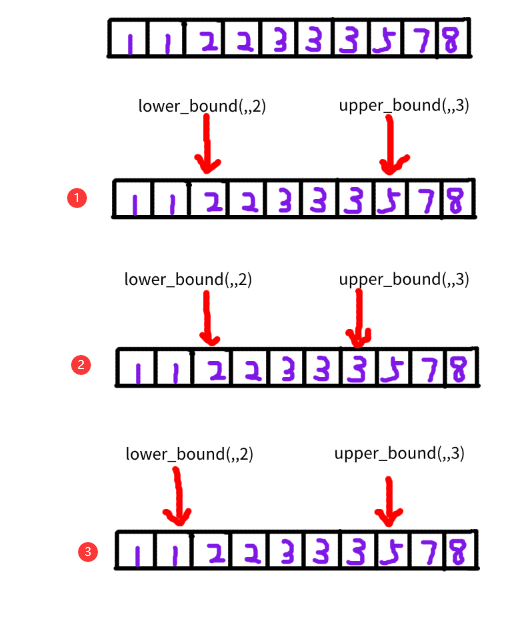对于一个有序数组，我们在实现 lower_bound() 函数和 upper_bound() 函数时可以让它返回指定的位置来确定取值区间，第①种情况就是标准函数库的实现方式，而第②种和第③种就是我第一印象中感觉应该对称的样子，这样看起来也没什么问题，下面具体来分析下后两种设计有哪些不好的地方。
具体分析
假如我们采用第②种实现方式，那么实现打印元素2和3的代码要写成下面这样：
#include <iostream>
#include <vector>
#include <algorithm>
using namespace std;

int main()
{
std::vector<int> v{1,1,2,2,3,3,3,5,7,8};
std::vector<int>::const_iterator itorLower = std::lower_bound(v.begin(), v.end(), 2);
std::vector<int>::const_iterator itorUpper = std::upper_bound(v.begin(), v.end(), 3);
while(true)
{
std::cout << *itorLower << std::endl;
if (itorLower == itorUpper)
break;
++itorLower;
}
return 0;
}

代码看起来还可以，打印完元素后判断到达了结尾直接跳出循环，但是如果要是数组中不包含元素2和3呢，那么也会打印出一个元素，还有可能导致程序崩溃。
如果我们采用第③种实现方式，那么实现打印元素2和3的代码要写成下面这样：
#include <iostream>
#include <vector>
#include <algorithm>
using namespace std;

int main()
{
std::vector<int> v{1,1,2,2,3,3,3,5,7,8};
std::vector<int>::const_iterator itorLower = std::lower_bound(v.begin(), v.end(), 2);
std::vector<int>::const_iterator itorUpper = std::upper_bound(v.begin(), v.end(), 3);
for(++itorLower; itorLower != itorUpper; ++itorLower)
{
std::cout << *itorLower << std::endl;
}
return 0;
}

这代码看起来简洁了很多，但是在循环开始前需要先调用 ++itorLower，因为第一个元素并不是需要找到的元素，所以要先跳过它，这样看来确实多做了一步操作，一开始就让 itorLow 指向第一个2就好了呀。
最终版本
当你尝试几种实现方式就会发现，还是标准库提供的这种方式使用起来更加方便，虽然采取的不是对称的方式，但是统一了存在查找元素和不存在查找元素的的情况，写出的代码也比较简洁，没有多余的步骤，代码如下：
#include <iostream>
#include <vector>
#include <algorithm>
using namespace std;

int main()
{
std::vector<int> v{1,1,2,2,3,3,3,5,7,8};
auto itorUpper = std::upper_bound(v.begin(), v.end(), 3);
for(auto itorLower = std::lower_bound(v.begin(), v.end(), 2); itorLower != itorUpper; ++itorLower)
{
std::cout << *itorLower << std::endl;
}
return 0;
}

总结
有些函数的实现方式和我们想象的并不一样，但是我们可以通过熟练使用来了解它为什么这样设计对称结构虽然是很美的，但是非对称的结构在编程中常常出现，同样有其美丽所在遇到类似的问题可以动笔画一画，列举出各种情况会有利于你做出正确的判断

==>> 反爬链接，请勿点击，原地爆炸，概不负责！<<==

有时会很焦虑，看到优秀的人比你还努力时总让人感到急迫，但是一味的忧患是无意义的，脚下迈出的每一步才是真真正正的前进，不要去忧虑可能根本就不会发生的事情，那样你会轻松许多

展开全文c++ 二分查找
• 文章目录前引upper_bound lower_bound算法理解my_upper_bound代码实现my_lower_bound代码实现相关习题博客链接 自写upper_bound lower_bound函数 前引 这部分今天在刷力扣专题的时候 就想到了自己实现upper_bound ...c++
• 在刷力扣上220. 存在重复元素 III的题目遇到了一个问题，原题目如下： 最开始的思路是维护一个最大长度为 k 的滑动窗口，窗口元素放在有序集合中，然后二分查找有序集合... auto p = lower_bound(st.begin(), st.e..
• 二分查找算法可以解决最简单的二分查找问题：a数组单调递增，并且其中...为了解决这些问题，C++ STL提供了两个特别好用的函数：lower_bound()和uppper_bound()。假设a是一个数组，n是数组长度，lower_bound(a, a+n...
• 前提：一个非降序列 lower_bound()函数使用： 参数： 1、数组元素的地址（起始...upper_bound()函数 返回值：返回第一个大于查找值的地址 应用例：三足鼎立问题 #include<iostream> #include<al...c++
• lower_bound( )和upper_bound( )都是利用封装的二分查找的方法在一个排好序的数组中进行查找的。 lower_bound (begin,end,num)是将一个排好序的[begin,end)区间中通过二分查找第一个大于等于num的数字，c++
• upper_bound( first , last , value ) 返回指向范围 [first, last) 中首个大于 value 的元素的迭代器，若找不到则返回 last 。 lower_bound( first , last , value ) 返回指向范围 [first, last) 中首个大于等于 ...
• lower_bound() 函数lower_bound()在**[lo, hi)进行二分查找，返回大于或等于**target的第一个元素的位置。如果所有元素都小于target，则返回hi. private int lower_bound(int[] nums, int lo, int hi, int target) {...
• lower_bound函数   函数lower_bound()在begin和end中的左闭右开区间进行二分查找，返回大于或等于val的第一个元素位置（迭代器）。如果所有元素都小于val，则返回last的位置。 注意STL中设计区间都是左闭右开，即...算法 二分查找
• 介绍结构体数组和包含结构体的vector怎么样使用lower_bound进行二分查找，upper_bound同理。 前提： lower_bound:返回数组中第一个大于等于该元素的下标，int aa = lower_bound(array,array+arrayLen,num) - array;...重复元素的二分查找
• 文章目录lower_bound函数和upper_bound函数的用法string中的find函数string中还有rfind()函数 lower_bound函数和upper_bound函数的用法 作用： 用于查找容器中大于等于某值的数，返回的这个数的指针。 vector容器内...c++
• lower_bound( )和upper_bound( )都是利用二分查找的方法在一个排好序的数组中进行查找的。在从小到大的排序数组中，lower_bound( begin,end,num)：从数组的begin位置到end-1位置二分查找第一个大于或等于num的数字，...
• 使用这两个函数都需要包含对应...upper_bound(查找的起始位置，查找的终止为止，需要查找的数 )是返回第一个大于等于需要查找的数的数的地址 注意上面的两种用法都需要原数组是小到大排列的有序数组 可以通过修改比c++
• lower_boundupper_bound尤其如此（ 没事手写了个二分查找，功能用法返回值和STL一毛一样（只是不能传函数，如果是对自己的结构体排序要定义小于运算符QAQ） 然后测试了一下对 intintint 排序，STL耗时是我的我5倍...二分查找 模板
• 递增数组 vector arr({1,2,3,4,5,6,8,9}); lower_bound lower_bound(arr.begin(), ...upper_bound(arr.begin(), arr.end(), num)；从数组的begin()位置到end()-1位置二分查找第一个大于num的数字，找到就返回该数字的c++
• } 综合应用 查询某个元素出现的次数 upper_bound - lower_bound #include using namespace std; int a; int main(){ int n; cin >> n; for(int i=0; i; i++) { cin >> a[i]; } sort(a,a+n); ...PAT
• upper_bound和lower_bound lower_bound:返回 upper_bound:
• 题意: 给一个长度为n的a数组和一个长度为m的b数组,问相邻的b数组元素中间最多有多少...lower_boundupper_bound的使用 代码实现: #include<bits/stdc++.h> using namespace std; typedef long long ll; const in
• lower_boundupper_bound 的用法（利用二分查找的方法在一个排好序的数组中进行查找的） 在从小到大的排序数组中 lower_bound(begin,end,val) 在begin和end中的前闭后开区间进行二分查找，返回大于或等于val的第...查找 STL
• C++ lower_bound()》一节中，系统地介绍了 lower_bound() 二分法查找函数的功能和用法，在此基础上，本节再讲解一个功能类似的查找函数，即 upper_bound() 函数。 upper_bound() 函数定义在头文件中，用于在指定范围...c++
• lower_bound()函数用于查找在指定区域内大于等于目标值的第一个元素。也就是说，使用该函数在指定范围内找某个目标值时，最终查找到的不一定是和目标值相等的元素，也可能是比目标值大的元素。 函数原型 lower_bound...
• C++STL常用操作之lower_boundupper_bound篇 简介： #include<algorithm> lower_bound(start,last,key)返回大于或者等于目标参数的第一个元素的位置 upper_bound(start,last,key)返回大于目标参数的第一个...算法 c++ 数据结构
• lower_bound查找序列中的第一个出现的值大于等于val的位置 int lower_bound(int *array,int l,int r,int targte){ while(l<=r){ int mid=(l+r)/2;...upper_bound返回的是最后一个大于val的位置 int upper_c++ 算法 stl
• lower_bound函数实现 函数功能是查找数组中第一个大于等于target的数的位置： int lower_bound(vector<int>& nums, int target) { int l = 0, r = nums.size(); while (l < r) { int mid = l + ...
• 在对 4 进行 upper_bound 时，输出结果是 12，因为在升序序列中 upper_bound 返回第一个大于 参数val 的 序列值的迭代器，不幸的是这个序列里找不到大于 4 的值，所以迭代器走到尽头也没有找到，于是返回了一个尾...c 迭代器 STL...